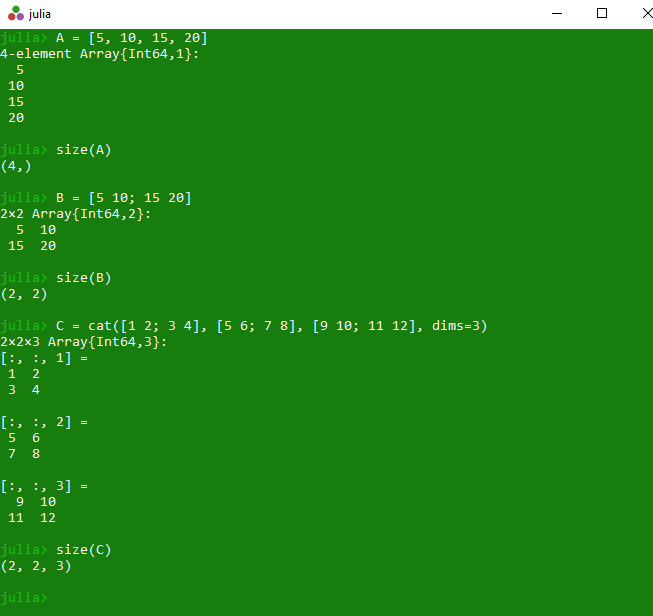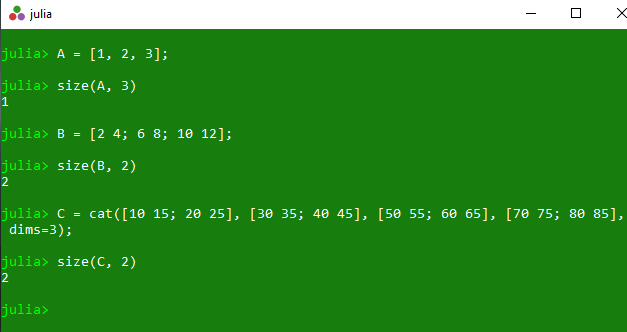# Get array dimensions and size of a dimension in Julia – size() Method

The `size()` is an inbuilt function in julia which is used to return a tuple containing the dimensions of the specified array. This returned tuple format is (a, b, c) where a is the rows, b is the columns and c is the height of the array.

Syntax:
size(A::AbstractArray)
or
size(A::AbstractArray, Dim)

Parameters:

• A: Specified array
• Dim: Specified dimension

Returns: It returns a tuple containing the dimensions of the specified array.

Example 1:

 `# Julia program to illustrate  ` `# the use of Array size() method ` ` `  `# Finding a tuple containing the dimension of ` `# the specified 1D array A. ` `A ``=` `[``5``, ``10``, ``15``, ``20``] ` `println(size(A)) ` ` `  `# Finding a tuple containing the dimension of ` `# the specified 2D array B of size 2*2 ` `B ``=` `[``5` `10``; ``15` `20``] ` `println(size(B)) ` ` `  `# Finding a tuple containing the dimension of ` `# the specified 3D array C of size 2*2*2 ` `C ``=` `cat([``1` `2``; ``3` `4``], [``5` `6``; ``7` `8``], [``9` `10``; ``11` `12``], dims``=``3``) ` `println(size(C)) `

Output:Example 2:

 `# Julia program to illustrate  ` `# the use of Array size() method ` `    `  `# Finding a tuple containing the dimension of ` `# the specified 1D array A. ` `A ``=` `[``1``, ``2``, ``3``]; ` `println(size(A, ``3``)) ` `    `  `# Finding a tuple containing the dimension of ` `# the specified 2D array B of size 3*2 ` `B ``=` `[``2` `4``; ``6` `8``; ``10` `12``]; ` `println(size(B, ``2``)) ` `    `  `# Finding a tuple containing the dimension of ` `# the specified 3D array C of size 2*2*4 ` `C ``=` `cat([``10` `15``; ``20` `25``], [``30` `35``; ``40` `45``], ` `        ``[``50` `55``; ``60` `65``], [``70` `75``; ``80` `85``], dims``=``3``); ` `println(size(C, ``2``)) `

Output:Whether you're preparing for your first job interview or aiming to upskill in this ever-evolving tech landscape, GeeksforGeeks Courses are your key to success. We provide top-quality content at affordable prices, all geared towards accelerating your growth in a time-bound manner. Join the millions we've already empowered, and we're here to do the same for you. Don't miss out - check it out now!

Previous
Next